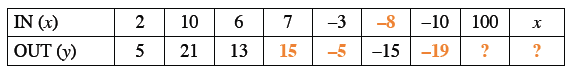### Home > CC3 > Chapter 3 > Lesson 3.1.2 > Problem3-13

3-13.

Copy and use your pattern skills to complete the table below. Homework Help ✎

 IN ($x$) $2$ $10$ $6$ $7$ $-3$ $-10$ $100$ $x$ OUT ($y$) $5$ $21$ $13$ $-15$1. Explain in words what is being done to the input value, $x$, to produce the output value, $y$.

Notice the output is two times the input plus one.

1. Write the process you described in part (a) in algebraic symbols.

Write part (a) in symbols remembering that input is $x$ and output is $y$.

$y=2x+1$